Function Repository Resource:

# EllipticExpand

Expand elliptic functions in an expression

Contributed by: Jan Mangaldan
 ResourceFunction["EllipticExpand"][expr] expands out elliptic functions in expr.

## Details and Options

ResourceFunction["EllipticExpand"] operates on both Jacobi and Weierstrass elliptic functions.
ResourceFunction["EllipticExpand"] splits up sums and integer multiples that appear in arguments of elliptic functions, using elliptic function identities when possible.
For expressions containing Jacobi elliptic functions, ResourceFunction["EllipticExpand"] produces an equivalent expression entirely in terms of JacobiSN, JacobiCN and JacobiDN.
ResourceFunction["EllipticExpand"] accepts the following options:
 "ExpandJacobi" True whether to expand Jacobi functions "ExpandNonElliptic" False whether to expand non-elliptic functions "ExpandWeierstrass" True whether to expand Weierstrass functions
With "ExpandNonElliptic"True, ResourceFunction["EllipticExpand"] expands out all instances of JacobiEpsilon (if "ExpandJacobi"True) and WeierstrassZeta (if "ExpandWeierstrass"True).
ResourceFunction["EllipticExpand"] automatically threads over lists, as well as equations, inequalities and logic functions.

## Examples

### Basic Examples (2)

Expand Jacobi elliptic function expressions:

 In:=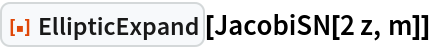Out=In:=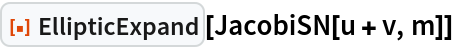Out=Expand Weierstrass elliptic function expressions:

 In:=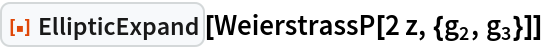Out=In:=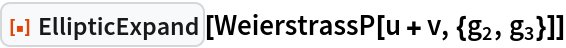Out=### Scope (4)

Expand Jacobi elliptic function expressions:

 In:=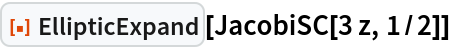Out=In:=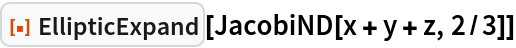Out=Expand Weierstrass elliptic function expressions:

 In:=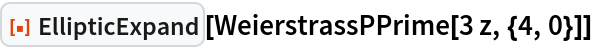Out=In:=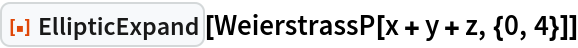Out=In:=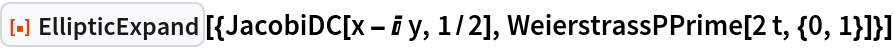Out=EllipticExpand threads over equations and inequalities:

 In:=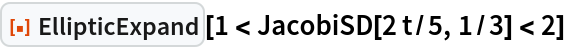Out=### Options (6)

#### ExpandJacobi (2)

With the setting "ExpandJacobi"True, Jacobi elliptic functions are expanded out:

 In:=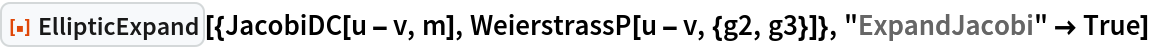Out=With the setting "ExpandJacobi"False, Jacobi elliptic functions are left untouched:

 In:=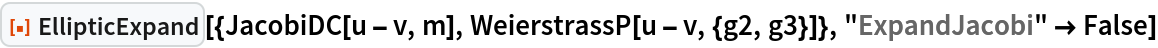Out=#### ExpandNonElliptic (2)

With the setting "ExpandNonElliptic"False, only Jacobi elliptic functions are expanded out:

 In:=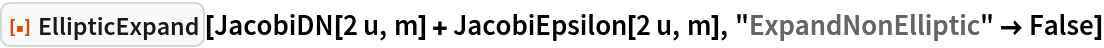Out=With the setting "ExpandNonElliptic"True, JacobiEpsilon expressions are also expanded out:

 In:=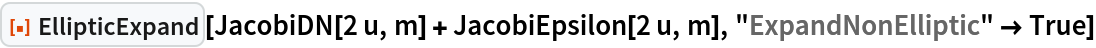Out=With the setting "ExpandNonElliptic"False, only Weierstrass elliptic functions are expanded out:

 In:=Out=With the setting "ExpandNonElliptic"True, WeierstrassZeta expressions are also expanded out:

 In:=Out=#### ExpandWeierstrass (2)

With the setting "ExpandWeierstrass"True, Weierstrass elliptic functions are expanded out:

 In:=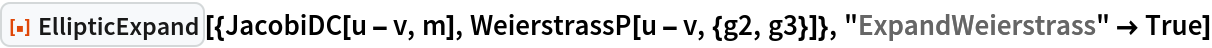Out=With the setting "ExpandWeierstrass"False, Weierstrass elliptic functions are left untouched:

 In:=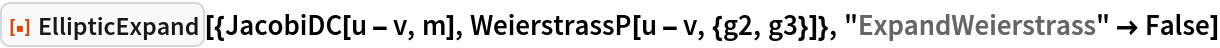Out=### Applications (2)

Find the addition law for InverseJacobiSN:

 In:=Out=Because of the multivaluedness of InverseJacobiSN, this law does not hold everywhere:

 In:=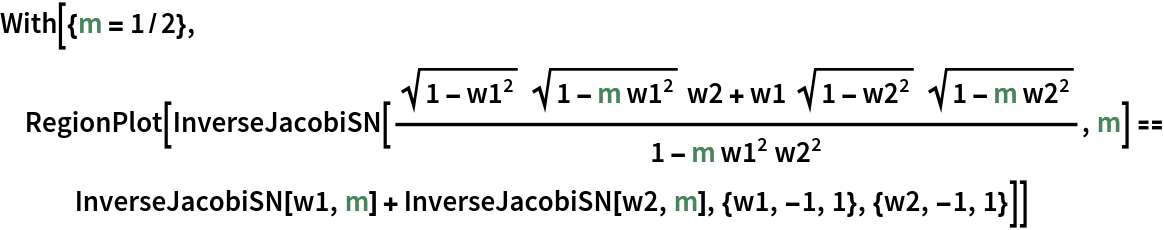Out=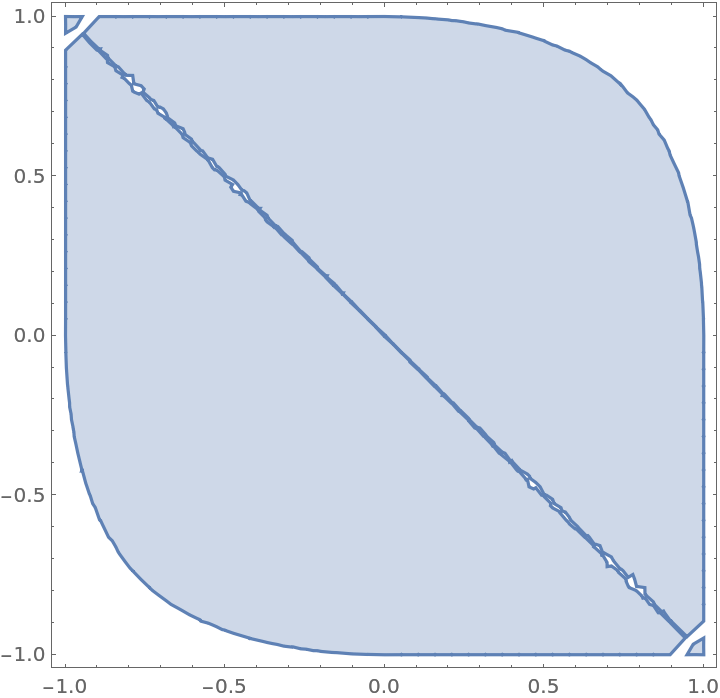In:=Out=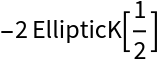## Version History

• 1.0.0 – 14 June 2021